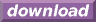#Calcute: freeware calculator

## Cube

The cube of a value is this value raised to the power of 3 (raised to the third power). Calcute implements this operation with the cube function. The same result will be obtained using the power operator ^ with the value of 3, or by multiplying the number by itself twice: cube(x) = x^3 = x*x*x.

```cube(2)
8
2^3
8
2*2*2
8
```